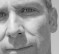## Implementing generic first order transfer functionStarted by 7 years ago6 replieslatest reply 7 years ago122 views
 Hi Can anyone tell me how to implement generic first order transfer function  a1s+a2/(b1s+b2) in Matlab meaning with for loop in the discrete form not using tf([a1 a2],[b1 b2]). For example if I want to implement a1/s I know I would do something like: value(n)=value(n-1)+a1*fx*Ts; But I am wondering how may I implement the generic (a1*s+a2)/(b1*s+b2) Thanks
[ - ]First, let's back up a bit.

You are not implementing the transfer function $$\frac{a_1}{s}$$.  To implement that transfer function you must find some analog electronics (or mechanics, or whatever) and implement the differential equation $$\frac{d}{dt} value \left ( t \right ) = a_1 x$$

What you are doing is to approximate the Laplace-domain transfer function $$\frac{a_1}{s}$$ with the z-domain transfer function $$\frac{a_1 T_s}{z - 1}$$.  Then you are implementing that approximation with the difference equation $$value_n = value_{n-1} + a_1 T_s x$$

So -- what are you trying to do?  Simulate a continuous-time system that incorporates the transfer function $$\frac{a_1 s + a_2}{b_1 s + b_2}$$?  Or do you want to approximate that transfer function in the z-domain and implement that?

[ - ]Great, answer I am familiar with bilinear transformation where s=2/T (z-1)/(z+1) so I can convert the s domain to z domain. I do not really know any technique that I can implement the actual transfer function in s domain in Matlab without z domain approximation, I will be excited to know if there is any method that can achieve this ?

I am also very interested to how you got a1*T/(z-1) what transformation did you use (is it like impulse invariance) I will be really appriciated if you can tell me more about approximation technique as well.

[ - ]What are you trying to DO???  The tree bark that you are describing seems off -- could you at least tell me what the tree is, if not give me an overview of the forest?

I got the transfer function in the z domain by looking at your difference equation (actually, I think I was wrong -- I think I meant $$\frac{a_1 T_z z}{z - 1}$$).

[ - ]I am trying to implement the transfer function in s domain in Matlab but not symbolically, that's all I am looking for. Any technique or method you know about that.

what are you trying to do?  Simulate a continuous-time system that ?  Or do you want to approximate that transfer function in the z-domain and implement that?

Either of them is fine as long as it produces accurate enough result.

[ - ]OK.  Give up on "implement the transfer function in s domain in Matlab".  That simply cannot be done as stated.

What you can do is express the associated differential equation in Matlab, and then use one of the solvers on Matlab to solve that expression for a specific input.  I'm not sure what's available in Matlab or it's various over-priced toolboxes, but there should be a simulator that deals with transfer functions or state-space linear systems, using all the nifty linear systems simplifications available, and if you're plopping a linear block into the middle of a system with nonlinearities, there's a nonlinear solver.

In either case, if you're doing it from the command line you need to be responsible for determining if you need one grand and glorious solution to solve (often necessary if there's feedback involved) or whether you can break it into pieces.  If you use their graphical interface (which name I cannot remember) then you can let it do the thinking for you, and if you're lucky it'll work (if you're not lucky then you'll end up doing more work than you would to just do it from the command line).

[ - ]